# Quantitative Hyperbolicity Estimates in One-Dimensional Dynamics

This page contains additional information and data referred to in the paper Quantitative hyperbolicity estimates in one-dimensional dynamics by Sarah Day, Hiroshi Kokubu, Stefano Luzzatto, Konstantin Mischaikow, Hiroe Oka, and Paweł Pilarczyk

A preprint of this paper can be downloaded here: [ PDF ] [ PS ]

Below is a list of files for download (please, see the description in the next sections). The zipped files should be uncompressed to empty subdirectories (folders), except for capd-capdDynSys-4.2.153.zip, which already includes a subfolder. Please, note that in Windows these files should be unzipped in the text mode to ensure the correct conversion of line endings (add the -a command-line switch in case of unzipping with the Info-ZIP software).

Note that all the files listed above may have been recently updated. Here are the original files that were published at the time of publication of the paper that are different from those listed above:

## Software

The software for computations described in the paper is written in the C++ programming language for optimal effectiveness and flexibility. Detailed documentation of the software has been prepared with Doxygen. Feel free to browse this documentation and learn about the details of how the software was programmed. The file unifexp-dox.zip contains the compressed version of this documentation which is available here for easy download for off-line browsing; please, unzip the contents of the archive into an empty directory, and open the file index.html.

The file unifexp-src.zip containing the source code of the software is available here for download under the terms of the GNU General Public License. It is prepared for the compilation with the GNU C++ compiler, either in GNU/Linux (or a similar system), or in Windows in the MSYS environment. A relatively recent version of the compiler is required to compile the code correctly. The provided makefile must be adjusted before the compilation, especially as far as the paths to the CAPD and CHomoP libraries are concerned (please, see the explanations in the file itself).

The software uses the Boost, CHomP and CAPD libraries which are publically available, the last two under the terms of the GNU General Public License. Please, refer to the websites of those libraries for the source code and compilation instructions. Note that the Boost library is often included in standard GNU/Linux distributions, so no installation might be necessary. This is not the case with the other libraries, though. Even worse, since those libraries are undergoing dynamic development, it may turn out that their interface may slightly change in the future versions, so a copy of compatible versions of both libraries is available for download from this page (please, see the previous section).

## Compilation of the Software

Step 1. Compilation of CHomP. This is my suggestion:

1. mkdir chomp
2. cd chomp
3. unzip -a ../chomp-lib.zip
4. make library
5. cd ..

Step 2. Compilation of CAPD. Since this library has many options, here is my suggestion for how to compile it. Note that it may be necessary to install the additional package pkg-config if it is not present in the system.

1. unzip -a capd-capdDynSys-4.2.153.zip
2. mkdir capd4
3. mkdir capd4_compil
4. cd capd4_compil
5. ../capd-capdDynSys-4.2.153/configure --prefix /home/your-dir/capd4 --with-filib=yes --with-mpfr=no --with-boost=/usr --without-gui
6. make
7. make install

Step 3. Compilation of the unifexp program. After having successfully compiled the CHomP and CAPD libraries, this is my suggestion how to proceed:

1. mkdir unifexp
2. cd unifexp
3. unzip -a ../unifexp-src.zip
4. make

Step 4. Testing the program. You can run a test computation to see if the program works:

• ./unifexp -p uniform -i --rigorous -k5001 -a1.83139961 -a1.83139961 --lambda --delta -L0 -L0 -f a00.dat --log a00.log --debug

Remark. It seems that the program unifexp does not compile with gcc 4.4.6 20120305 (Red Hat 4.4.6-4) due to a problem with the power function in CAPD. The same with gcc (Debian 4.4.5-8) 4.4.5. If you encounter this kind of a problem, please, try using a more recent version of the GCC compiler.

Remark for Mac users. I tested this software on a Mac with OS X El Capitan Version 10.11.6 and GNU C++ compiler 7.2.0 provided by MacPorts. For compiling the CHomP library, I used the command

• make target=macports
While compiling the CAPD library, I modified the configure command to
• export CC=gcc-mp-7 -m64
• export CXX=g++-mp-7 -m64
• ../capd-capdDynSys-4.2.153/configure --prefix /Users/pawel.pilarczyk/capd4 --with-filib=yes --with-mpfr=no --with-boost=/usr --without-gui CXX="g++-mp-7 -m64" CC="gcc-mp-7 -m64" CXXFLAGS="-O2 -Wall"
I also compiled the unifexp program with the command
• make cxx="g++-mp-7 -m64"

## Results of Computations

The raw data with the results of computations used to create the figures published in the paper, and also with some other results, can be downloaded below. All these results were produced by the software described in the previous section and are encoded in text files whose lines contain the following items as a space-separated list:

• the asterisk that indicates the beginning of a data set (comments are preceded with the semicolon)
• the identifier of the data piece in the format level:number (e.g., 4:12)
• the left end of the parameter interval (minimal parameter value)
• the right end of the parameter interval (maximal parameter value)
• the total number of intervals that cover both Δ and the remainder of I
• the diameter δ of the critical neighborhood
• the computed expansion exponent λ
• the computed value of log C (0 if not computed)
• the computed value of λ0 (0 if not computed)
• the computation time measured in seconds
The raw data is contained in files named a01.dat, a02.dat, etc., which are together compressed in the single file unifexp-dat.zip for easy download. Brief descriptions of the computations are available below together with some PDF files with figures produced from this data with gnuplot. All the computations were conducted with the switches -i and --rigorous of the program to ensure rigorous computations with interval arithmetic, and also taking entire (sub)intervals of a where a was varying, with the exception for a few series of computations marked as non-rigorous. The range of each variable (delta, K, or a) which was changing in each series of computations was divided into 4096 almost equal parts. In case of delta and K, almost equally spaced single values were take for each computation. In case of a, entire intervals were taken in order to ensure complete coverage of the interval [1.7,2].

 specifications graphs a01.dat K=5000delta: 0.005-0.5a=2.0lambda as a function of-log(delta)computation timeas a function of -log(delta) a02.dat K: 100-7900delta=0.01a=2.0Floyd-Warshalllambda as a function of Kcomputation timeas a function of K a03.dat K: 100-7900delta=0.01a=2.0Johnson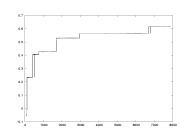lambda as a function of K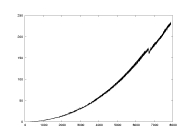computation timeas a function of K a04.dat K: 100-7900delta=0.01a=2.0critical partition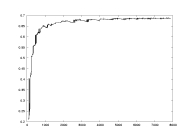lambda as a function of K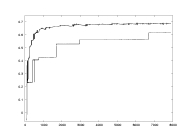comparison of lambda computed with critical vs. uniform partition a05.dat K=5000delta=0.1a: 1.7-2.0critical partition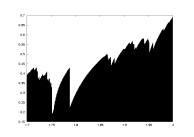lambda as a function of alambda0 as a function of a a06.dat K=5000delta=0.1a: 1.7-2.0uniform partition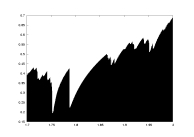lambda as a function of alambda0 as a function of a a07.dat K=5000delta=0.1a: 1.7-2.0derivative partition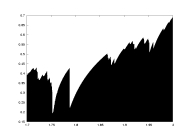lambda as a function of a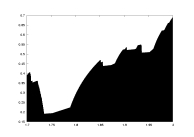lambda0 as a function of a a08.dat K=5000delta=0.001a: 1.7-2.0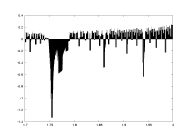lambda as a function of acomputation time as a function of a a09.dat K=5000delta=0.01a: 1.7-2.0lambda as a function of a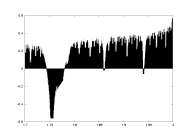lambda0 as a function of a a10.dat K=5000delta minlambda>0a: 1.7-2.0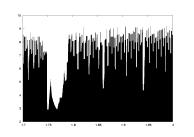-log(delta) as a function of a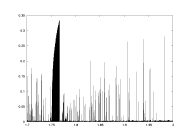lambda as a function of a a11.dat K=5000delta minlambda>0.1a: 1.7-2.0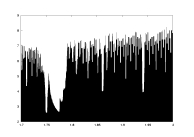-log(delta) as a function of alambda as a function of a a12.dat K=5000delta minlambda0>0a: 1.7-2.0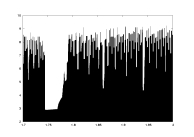-log(delta) as a function of alambda0 as a function of a a13.dat K=5000delta minlambda0>0.1a: 1.7-2.0-log(delta) as a function of alambda0 as a function of a a14.dat K: 100-7900delta=0.01a=2.0(computinglambda only)lambda as a function of Kcomputation timeas a function of K a15.dat K=5000delta=0.01a: -2.0-2.0cubic mapnon-rigorous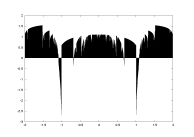lambda as a function of a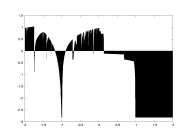lambda0 as a function of a a16.dat K=5000delta=0.05a: 0.67-1.0unimodal mapwith gamma=1.5non-rigorous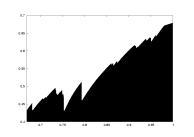lambda as a function of a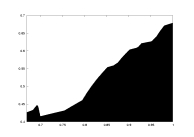lambda0 as a function of a a17.dat K=5000delta=0.05a: 0.9-1.0unimodal mapwith gamma=2.5non-rigorous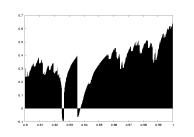lambda as a function of alambda0 as a function of a a18.dat K=4000delta=0.02a: 1.5-2.0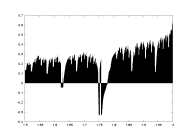lambda as a function of a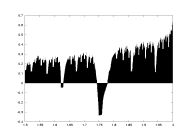lambda0 as a function of a a19.dat K: 100-7900delta=0.01a=2.0derivative partition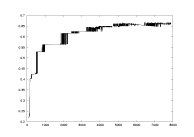lambda as a function of K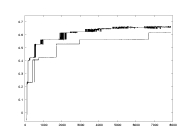comparison of lambda computed with derivative vs. uniform partition

The figures in the paper were generated using the following data:

• Figure 3 - a01.dat
• Figure 4 - a02.dat
• Figure 5 - a02.dat and a04.dat
• Figure 6 - a02.dat and a03.dat
• Figure 7 - a10.dat
• Figure 8 - a09.dat
• Figure 9 - a10.dat